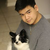0

# Find should format special characters when Regex is enabled

Victor Thanh Vo 2 года назад

Using Ctrl+F (Find shortcut) on highlighted text should automatically format the search text to escape RegEx special characters when RegEx is enabled.

Use case:

As a developer trying to find a group of function calls, I want to be able to highlight a block of code, and use the Find shortcut to navigate around my code, whether regex is enabled or disabled.

Currently, I get no results because the parentheses, plus-signs, periods, brackets, stars, etc are used for regex instead of being matched, either getting no results or reporting regex errors. My expectation is that Highlight, Ctrl+F should not have Find errors.

In the specific use case below, I want the regex to be on, otherwise I can't find the test2's CHECK_EQUAL group. I can provide more feedback/requirements if this idea is interesting.

Current find txt:
"
CHECK_EQUAL(for(bar(blah1)), for(bar(bleh1)))
CHECK_EQUAL(for(bar(blah2)), for(bar(bleh2)))
CHECK_EQUAL(for(bar(blah3)), for(bar(bleh3)))
CHECK_EQUAL(for(bar(blah4)), for(bar(bleh4)))
"
Desired find txt:
"
CHECK_EQUAL\(for\(bar\(blah1\)\), for\(bar\(bleh1\)\)\)
CHECK_EQUAL\(for\(bar\(blah2\)\), for\(bar\(bleh2\)\)\)
CHECK_EQUAL\(for\(bar\(blah3\)\), for\(bar\(bleh3\)\)\)
CHECK_EQUAL\(for\(bar\(blah4\)\), for\(bar\(bleh4\)\)\)
"
Find txt after user input:
"
CHECK_EQUAL\(for\(bar\(blah1\)\), for\(bar\(bleh1\)\)\)
CHECK_EQUAL\(for\(bar\(blah2\)\), for\(bar\(bleh2\)\)\)
CHECK_EQUAL\(for\(bar\(blah3\)\), for\(bar\(bleh3\)\)\)
CHECK_EQUAL\(for\(bar\(blah4\)\), for\(bar\((not)?bleh4\)\)\)
"

File content:
"
TEST(Test_group1, test1)
{
CHECK_EQUAL(for(bar(blah1)), for(bar(bleh1)))
CHECK_EQUAL(for(bar(blah2)), for(bar(bleh2)))
CHECK_EQUAL(for(bar(blah3)), for(bar(bleh3)))
CHECK_EQUAL(for(bar(blah4)), for(bar(bleh4)))
}

TEST(Test_group1, test2)
{
CHECK_EQUAL(for(bar(blah1)), for(bar(bleh1)))
CHECK_EQUAL(for(bar(blah2)), for(bar(bleh2)))
CHECK_EQUAL(for(bar(blah3)), for(bar(bleh3)))
CHECK_EQUAL(for(bar(blah4)), for(bar(notbleh4)))
}
"

Сервис поддержки клиентов работает на платформе UserEcho